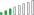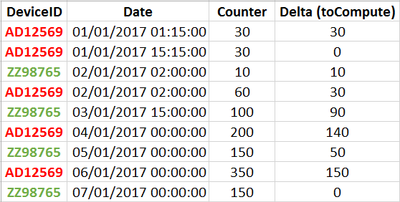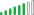cancel
Showing results for
Did you mean:

Fabric is Generally Available. Browse Fabric Presentations. Work towards your Fabric certification with the Cloud Skills Challenge.Helper I

## Delta calculation in PBI and counter

Hi everyone,

I have a device with one button communicating by Sigfox.
Each 15 minutes, I receive a new line in my DB with the number of press on the button (counter !).
I received a message with date / number of press without the delta because it's a counter.

Example
01-01-2017 / 10 =>it means 10 press on this date
02-01-2017 / 15 => it means 5 press (15 - 10)
03-01-2017 / 25 => it means 10 press (25 - 15)
...

Have you got an idea or a simple method to allow me to have the number of press for each day using only PowerBI ?
Other point to mention is that this counter restart to 0 when it arrives at 4095...

Thank you for your ideasCheers,
Michaël

3 ACCEPTED SOLUTIONSResolver III
```Delta =
VAR EarlierDate = CALCULATE(MAX(TEST[date]), FILTER(TEST, TEST[Date] < EARLIER(TEST[date])))
VAR EarlierCumulativeTotal = CALCULATE(SUM(TEST[Cumulative Total]), ALL(TEST), TEST[date] = EarlierDate)
VAR DeltaTemp = TEST[Cumulative Total] - EarlierCumulativeTotal
RETURN IF(DeltaTemp>0, DeltaTemp, (4095-EarlierCumulativeTotal)+TEST[Cumulative Total])```Helper I

It works like a charm 🙂

Many thanksResolver III

Try this:

```DeviceDelta =
VAR EarlierDate = CALCULATE(MAX(TEST[date]), FILTER(TEST, TEST[Date] < EARLIER(TEST[date]) && TEST[DeviceID] = EARLIER(TEST[DeviceID])))
VAR EarlierCumulativeTotal = CALCULATE(SUM(TEST[Cumulative Total]), ALL(TEST), TEST[date] = EarlierDate,TEST[DeviceID] = EARLIER(TEST[DeviceID]))
VAR DeltaTemp = TEST[Cumulative Total] - EarlierCumulativeTotal
RETURN IF(DeltaTemp>=0, DeltaTemp, (4095-EarlierCumulativeTotal)+TEST[Cumulative Total])```
9 REPLIES 9Resolver III

 date Cumulative Total 1/1/2017 10 2/1/2017 15 3/1/2017 25

You can create a calculated column to get the deltas as such:

```Delta =
VAR EarlierDate = CALCULATE(MAX(TEST[date]),FILTER(TEST, TEST[Date] < EARLIER(TEST[date])))
RETURN TEST[Cumulative Total] - CALCULATE(SUM(TEST[Cumulative Total]), ALL(TEST), TEST[date] = EarlierDate)```Resolver III

For the reset at 4095, you could do something like this:

```Delta =
VAR EarlierDate = CALCULATE(MAX(TEST[date]), FILTER(TEST, TEST[Date] < EARLIER(TEST[date])))
VAR EarlierCumulativeTotal = CALCULATE(SUM(TEST[Cumulative Total]), ALL(TEST), TEST[date] = EarlierDate)
VAR DeltaTemp = TEST[Cumulative Total] - EarlierCumulativeTotal
RETURN IF(DeltaTemp>0, DeltaTemp, TEST[Cumulative Total])```

This would result in the following if you have data where the amount resets:Helper I

Thank you to both of you.

For the moment, I choose the solution @bblais.

Your solution is partially good because in your example you missed "8 messages".

So for 5/1/2017, the delta should be 18 instead of 10 (8 messages between 4087 and 4095 + 10).

Any idea using a simple way ?Resolver III
```Delta =
VAR EarlierDate = CALCULATE(MAX(TEST[date]), FILTER(TEST, TEST[Date] < EARLIER(TEST[date])))
VAR EarlierCumulativeTotal = CALCULATE(SUM(TEST[Cumulative Total]), ALL(TEST), TEST[date] = EarlierDate)
VAR DeltaTemp = TEST[Cumulative Total] - EarlierCumulativeTotal
RETURN IF(DeltaTemp>0, DeltaTemp, (4095-EarlierCumulativeTotal)+TEST[Cumulative Total])```Helper I

Still using line code, is it possible to indicate the device (first column) where to make the computation (according to the "date") and also take into account that a new line can be added for a device with a delta = 0 (because of no change between two receptions)?

Example of data I can receive (the last column indicates the value I want to find using computation)Resolver III

Try this:

```DeviceDelta =
VAR EarlierDate = CALCULATE(MAX(TEST[date]), FILTER(TEST, TEST[Date] < EARLIER(TEST[date]) && TEST[DeviceID] = EARLIER(TEST[DeviceID])))
VAR EarlierCumulativeTotal = CALCULATE(SUM(TEST[Cumulative Total]), ALL(TEST), TEST[date] = EarlierDate,TEST[DeviceID] = EARLIER(TEST[DeviceID]))
VAR DeltaTemp = TEST[Cumulative Total] - EarlierCumulativeTotal
RETURN IF(DeltaTemp>=0, DeltaTemp, (4095-EarlierCumulativeTotal)+TEST[Cumulative Total])```Helper I

It works like a charm too 🙂

Thanks !Helper I

It works like a charm 🙂

Many thanksSolution Supplier

Assuming the source table is called Data and the columns are named Date and Cumulated, here is my suggestion for your offsetting problem:

`let Source = Data, #"Sorted Rows" = Table.Sort(Source,{{"Date", Order.Ascending}}), #"Added Index" = Table.AddIndexColumn(#"Sorted Rows", "Index", 0, 1), Previous = {0} & List.RemoveLastN(#"Added Index"[Cumulated],1), Columns = Table.ToColumns( #"Added Index" ) & { Previous }, ColumnNames = Table.ColumnNames( #"Added Index" ) & {"Previous"}, Table = Table.FromColumns( Columns, ColumnNames)in Table`

This will add a new column with the value of Cumulated in the previous row.

From there, you should be able to calculate the delta (also applying the logic to handle resets).Announcements#### Power BI Monthly Update - November 2023

Check out the November 2023 Power BI update to learn about new features.#### Fabric Community News unified experience

Read the latest Fabric Community announcements, including updates on Power BI, Synapse, Data Factory and Data Activator.#### Exclusive opportunity for Women!

Join us for a free, hands-on Microsoft workshop led by women trainers for women where you will learn how to build a Dashboard in a Day!#### The largest Power BI and Fabric virtual conference

130+ sessions, 130+ speakers, Product managers, MVPs, and experts. All about Power BI and Fabric. Attend online or watch the recordings.Top Solution Authors
Top Kudoed Authors
Users online (4,911)